1596285180

# Decision Trees Classifier

Both of `Regression Trees` and `Classification Trees` are a part of `CART (Classification And Regression Tree) Algorithm`. As we mentioned in Regression Trees article, tree is composed of 3-major parts; root-node, decision-node and terminal/leaf-node.

The criteria used here in node splitting differs from that being used in Regression Trees. As before we will run our example and then learn how the model is being trained.

There are three commonly measures are used in the attribute selection `Gini impurity` measure, is the one used by `CART` classifier. For more information on these, see Wikipedia.

# Data set being used is `iris` data set

``````import numpy as np
import pandas as pd
from sklearn.tree import DecisionTreeClassifier
from sklearn.tree import export_graphviz
from six import StringIO
from IPython.display import Image
# pip/conda install pydotplus
import pydotplus
from sklearn import datasets
xList = iris.data  # Data will be loaded as an array
labels = iris.target
dataset = pd.DataFrame(data=xList,
columns=iris.feature_names)
dataset['target'] = labels
targetNames = iris.target_names
print(targetNames)
print(dataset)
``````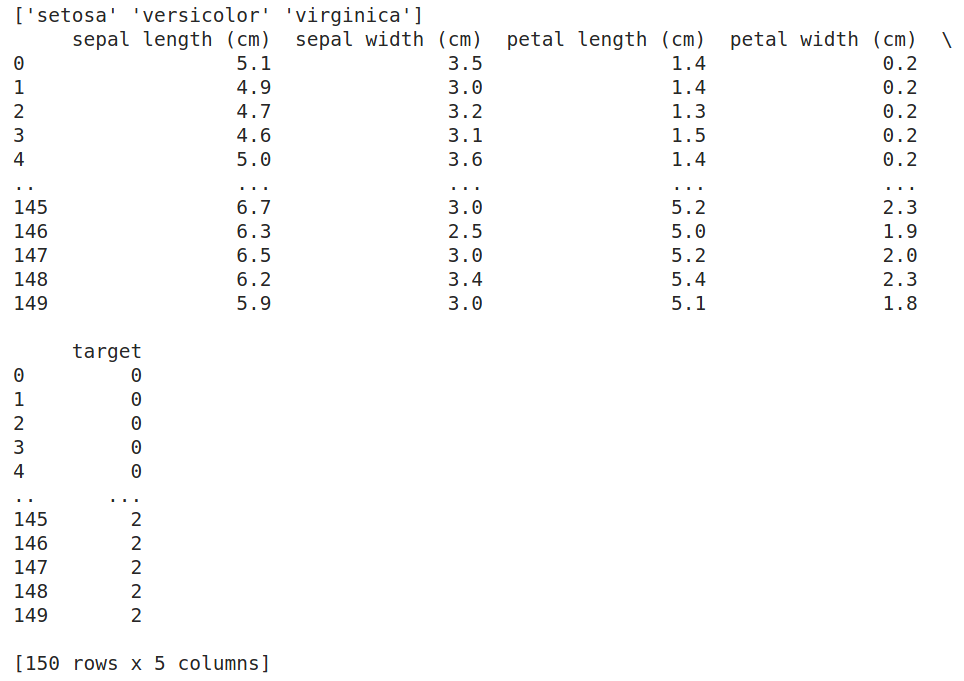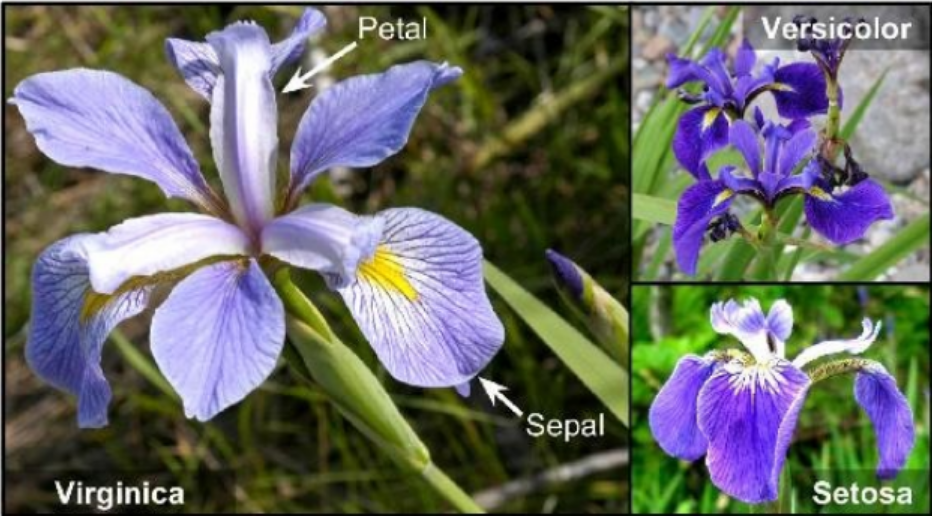Iris Flowers

# How a Binary Decision Tree Generates Predictions

When an observation or row is passed to a non-terminal node, the row answers the node’s question. If it answers yes, the row of attributes is passed to the leaf node below and to the left of the current node. If the row answers no, the row of attributes is passed to the leaf node below and to the right of the current node. The process continues recursively until the row arrives at a terminal (that is, leaf) node where a prediction value is assigned to the row. The value assigned by the leaf node is the mean of the outcomes of the all the training observations that wound up in the leaf node.

# How to split the nodes

Classification trees split a node into two sub-nodes. Splitting into sub-nodes will increase the homogeneity of resultant sub-nodes. In other words, we can say that the purity of the node increases with respect to the target variable. The decision tree splits the nodes on all available variables and then selects the split which results in most homogeneous/pure sub-nodes.

There are major measures being used to determine which attribute/feature is used for splitting and which value within this attribute we will start with. Some of these measures are:

• Gini index (Default in SciKit Learn Classifier).
• Entropy.
• Information gain.

We will start with `Gini index` measure and try to understand it

# Gini Index

Gini index is an impurity measure used to evaluate splits in the dataset. It is calculated by getting the sum of the squared probabilities of each class (target-class) within a certain attribute/feature then benig subtacted from one.

#machine-learning #mls #decision-tree #decision-tree-classifier #classification #deep learning

## Buddha Community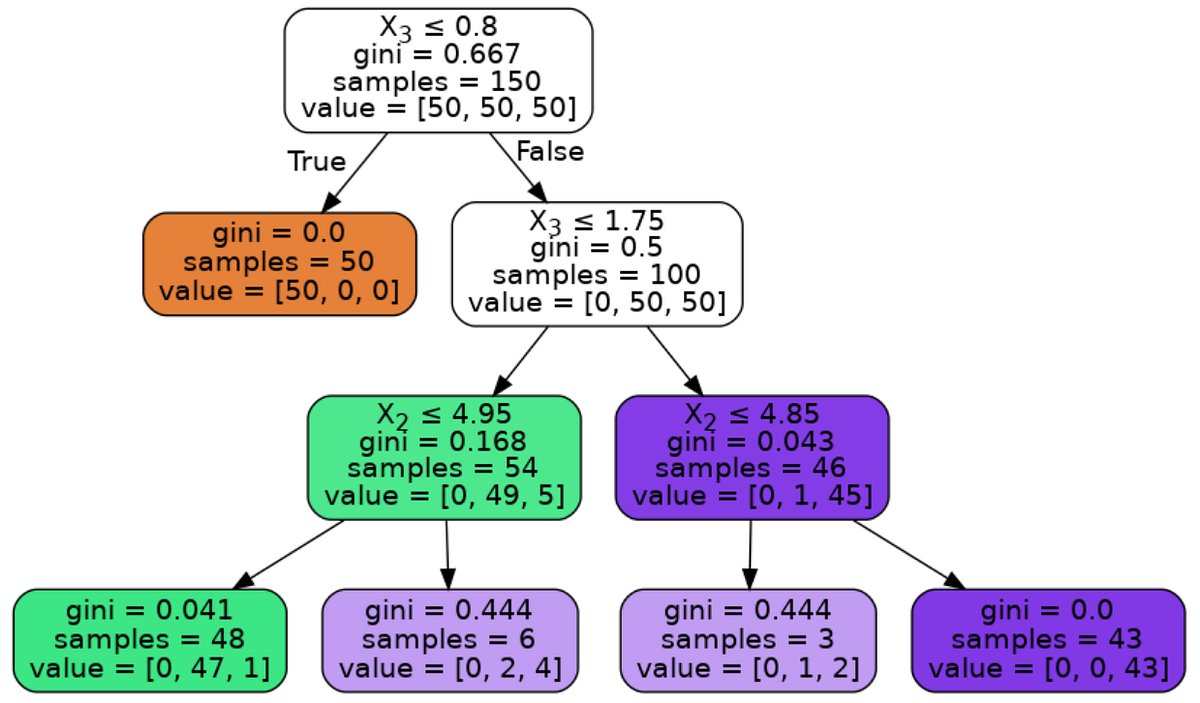1596286260

## Decision Tree -Classification

Decision Tree is one of the most widely used machine learning algorithm. It is a supervised learning algorithm that can perform both classification and regression operations.

As the name suggest, it uses a tree like structure to make decisions on the given dataset. Each internal node of the tree represent a “decision” taken by the model based on any of our attributes. From this decision, we can seperate classes or predict values.

Let’s look at both classification and regression operations one by one.

# Classification

In Classification, each leaf node of our decision tree represents a **class **based on the decisions we make on attributes at internal nodes.

To understand it more properly let us look at an example. I have used the Iris Flower Dataset from sklearn library. You can refer the complete code on Github — Here.

Image for post

A node’s samples attribute counts how many training instances it applies to. For example, 100 training instances have a petal width ≤ 2.45 cm .

A node’s value attribute tells you how many training instances of each class this node applies to. For example, the bottom-right node applies to 0 Iris-Setosa, 0 Iris- Versicolor, and 43 Iris-Virginica.

And a node’s gini attribute measures its impurity: a node is “pure” (gini=0) if all training instances it applies to belong to the same class. For example, since the depth-1 left node applies only to Iris-Setosa training instances, it is pure and its gini score is 0.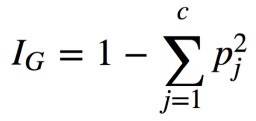Gini Impurity Formula

where, pⱼ is the ratio of instances of class j among all training instances at that node.

Based on the decisions made at each internal node, we can sketch decision boundaries to visualize the model.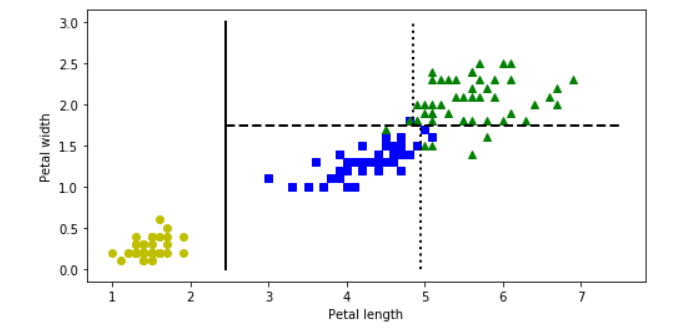But how do we find these boundaries ?

We use Classification And Regression Tree (CART) to find these boundaries.

CART is a simple algorithm that finds an attribute _k _and a threshold _t_ₖat which we get a purest subset. Purest subset means that either of the subsets contain maximum proportion of one particular class. For example, left node at depth-2 has maximum proportion of Iris-Versicolor class i.e 49 of 54. In the _CART cost function, _we split the training set in such a way that we get minimum gini impurity.The CART cost function is given as: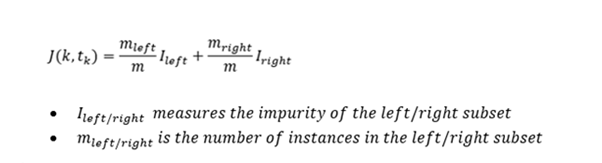After successfully splitting the dataset into two, we repeat the process on either sides of the tree.

We can directly implement Decision tree with the help of Scikit learn library. It has a class called DecisionTreeClassifier which trains the model for us directly and we can adjust the hyperparameters as per our requirements.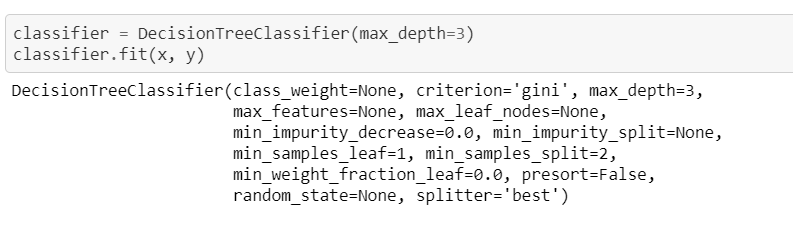#machine-learning #decision-tree #decision-tree-classifier #decision-tree-regressor #deep learning1596285180

## Decision Trees Classifier

Both of `Regression Trees` and `Classification Trees` are a part of `CART (Classification And Regression Tree) Algorithm`. As we mentioned in Regression Trees article, tree is composed of 3-major parts; root-node, decision-node and terminal/leaf-node.

The criteria used here in node splitting differs from that being used in Regression Trees. As before we will run our example and then learn how the model is being trained.

There are three commonly measures are used in the attribute selection `Gini impurity` measure, is the one used by `CART` classifier. For more information on these, see Wikipedia.

# Data set being used is `iris` data set

``````import numpy as np
import pandas as pd
from sklearn.tree import DecisionTreeClassifier
from sklearn.tree import export_graphviz
from six import StringIO
from IPython.display import Image
# pip/conda install pydotplus
import pydotplus
from sklearn import datasets
xList = iris.data  # Data will be loaded as an array
labels = iris.target
dataset = pd.DataFrame(data=xList,
columns=iris.feature_names)
dataset['target'] = labels
targetNames = iris.target_names
print(targetNames)
print(dataset)
``````Iris Flowers

# How a Binary Decision Tree Generates Predictions

When an observation or row is passed to a non-terminal node, the row answers the node’s question. If it answers yes, the row of attributes is passed to the leaf node below and to the left of the current node. If the row answers no, the row of attributes is passed to the leaf node below and to the right of the current node. The process continues recursively until the row arrives at a terminal (that is, leaf) node where a prediction value is assigned to the row. The value assigned by the leaf node is the mean of the outcomes of the all the training observations that wound up in the leaf node.

# How to split the nodes

Classification trees split a node into two sub-nodes. Splitting into sub-nodes will increase the homogeneity of resultant sub-nodes. In other words, we can say that the purity of the node increases with respect to the target variable. The decision tree splits the nodes on all available variables and then selects the split which results in most homogeneous/pure sub-nodes.

There are major measures being used to determine which attribute/feature is used for splitting and which value within this attribute we will start with. Some of these measures are:

• Gini index (Default in SciKit Learn Classifier).
• Entropy.
• Information gain.

We will start with `Gini index` measure and try to understand it

# Gini Index

Gini index is an impurity measure used to evaluate splits in the dataset. It is calculated by getting the sum of the squared probabilities of each class (target-class) within a certain attribute/feature then benig subtacted from one.

#machine-learning #mls #decision-tree #decision-tree-classifier #classification #deep learning1598063220

## Maths behind Decision Tree Classifier

Before we see the python implementation of the decision tree. Let’s first understand the math behind the decision tree classification. We will see how all the above-mentioned terms are used for splitting.

We will use a simple dataset which contains information about students from different classes and gender and see whether they stay in the school’s hostel or not.

This is how our data set looks like :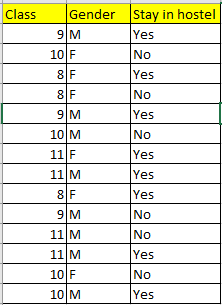Let’s try and understand how the root node is selected by calcualting gini impurity. We will use the above mentioned data.

We have two features which we can use for nodes: “Class” and “Gender”. We will calculate gini impurity for each of the features and then select that feature which has least gini impurity.

Let’s review the formula for calculating ginni impurity: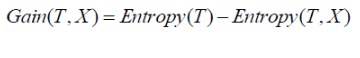Let’s start with class, we will try to gini impurity for all different values in “class”.

#data-science #machine-learning #decision-tree-classifier #decision-tree #deep learning1602997200

## Decision Tree Intuition

Decision Trees are easy & Simple to implement & interpreted. Decision Tree is a diagram (flow) that is used to predict the course of action or a probability. Each branch of the decision tree represents an outcome or decision or a reaction. Decision Trees can be implemented in a variety of situations from personal to complex situations. The sequence of steps will give a better understanding easily.

In Programming, we regularly use If-else conditions, even the Decision Tree working process is similar to an If-else condition.

## Let’s see how a decision Tree Looks like

The below tree shows a simple implementation of different nodes in the decision tree.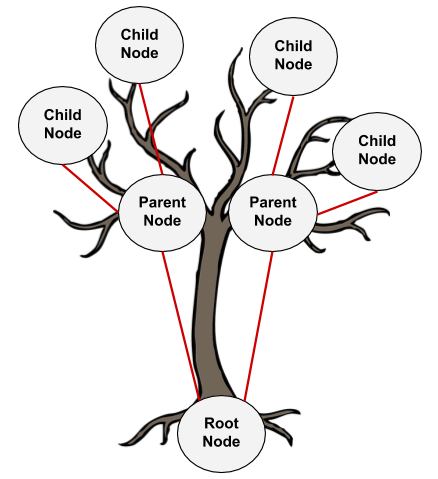1. **Root Node: **Root Node is a top node with the base feature.
2. **Parent Node: **Nodes that get their origin from a root node or this can be represented as a decision node where the decision of Yes/No or True/False or prediction turn happens.
3. **Child Node: **these nodes get their origin from a parent node. If the decision made from a parent node is not satisfactory then these nodes will be created. Until we arrive at the final node where we have pure domination in a class (Yes/No) means that until we arrive at the leaf node.
4. **Leaf Node: **Can also be called a terminal Node or a final decision node where we will conclude.

#gini-index #entropy #decision-tree #decision-tree-classifier #machine-learning1596960480

## Decision Tree (Classification) with sklearn

A classification tree is very alike to a regression tree, besides that it is

used to predict a qualitative response rather than a quantitative one. classification tree, we predict that each observation belongs to the most ordinarily occurring class of training observations in the region to which it belongs.

In the classification, RSS cannot be used for making the binary splits. An alternative to RSS is the classification error rate. we assign an observation in a given region to the most commonly occurring class of training observations in that region,** the classification error rate is simply the fraction of the training observations in that region that do not belong to the most common class:**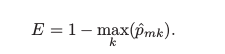p̂mk = proportion of training observations in the mth region that is from the kth class.

classification error is not good enough for tree-growing, and in practice, two other measures are favoured.

1. Gini index is defined by a measure of total variance across the K classes. It takes on a small value if all of the p̂mk ’s are close to 0 or 1. Therefore it is referred to as a **measure of node purity **— a small value shows that a node contains predominantly observations from a single class.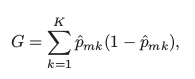2.** Cross-Entropy **is given by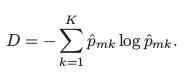Since, 0 ≤ p̂mk ≤ 1, it follows that 0 ≤ −p̂mk log p̂mk. The cross-entropy will take on a value near zero if the p̂mk ’s are all near 0 or near 1. Therefore, the cross-entropy will take on a small value if the mth node is pure. It turns out that the Gini index and the cross-entropy are quite similar numerically.

Two approaches are more sensitive to node purity than is the classification error rate. Any of these three approaches might be used when pruning the tree, but the classification error rate is preferable if the prediction accuracy of the final pruned tree is the goal.

Decision tree classifier using sklearn

To implement the decision tree classifier, we’re going to use scikit-learn, and we’ll import our ‘’DecisionTreeClassifier’’ from sklearn.tree

``````import numpy as np
import pandas as pd
import matplotlib.pyplot as plt
from sklearn import datasets
from sklearn.tree import DecisionTreeClassifier
``````